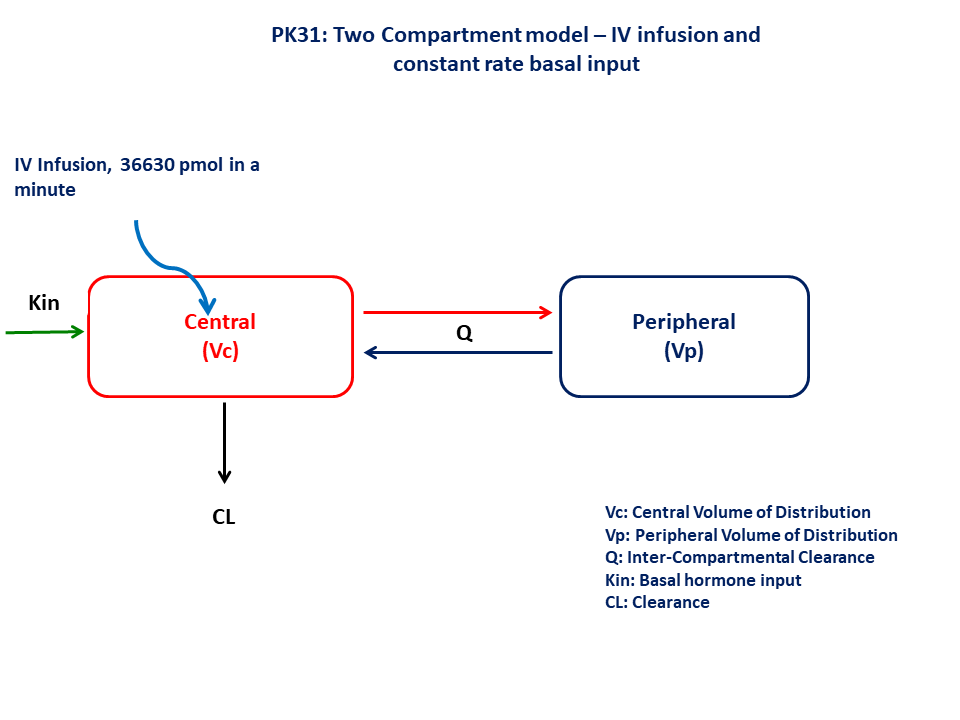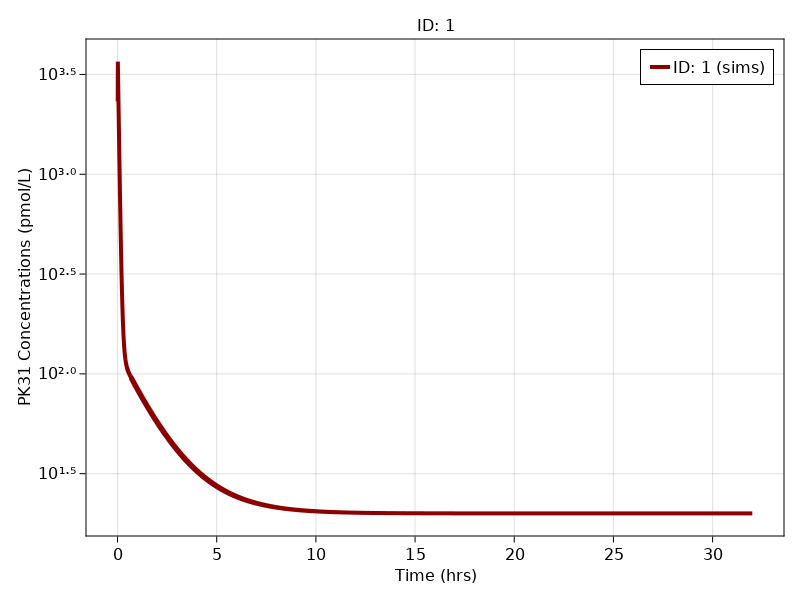# Exercise - PK31 Turnover II - Intravenous dosing of hormone

### Background

• Structural model - Two compartment with additional input for basal hormone synthesis in the central compartment

• Route of administration - IV infusion (1 minute)

• Dosage Regimen - 36,630 pmol

• Number of Subjects - 1### Learning Outcome

In this model, you will learn how to build a two compartment with additional input for basal hormone level. This model will help to simulate the plasma concentration profile after IV administration considering basal hormone input.

### Objectives

• To analyze the intravenous datasets with parallel turnover

• To write multi-compartment model in terms of differential equations

### Libraries

Call the "necessary" libraries to get start.

using Random
using Pumas
using PumasUtilities
using CairoMakie


### Model

In this one compartment model PK31, we administer IV infusion dose to central compartment.

pk_31           = @model begin
desc        = "Two Compartment Model"
timeu       = u"hr"
end

@param begin
"Basal hormonal input (pmol/hr)"
tvkin       ∈ RealDomain(lower=0)
"Central Volume of Distribution (L)"
tvvc        ∈ RealDomain(lower=0)
"Clearance (L/hr)"
tvcl        ∈ RealDomain(lower=0)
"Intercompartmental Clearance (L/hr)"
tvq         ∈ RealDomain(lower=0)
"Peripheral Volume of Distribution (L)"
tvvp        ∈ RealDomain(lower=0)
Ω           ∈ PDiagDomain(5)
"Proportional RUV"
σ²_prop     ∈ RealDomain(lower=0)

end

@random begin
η           ~ MvNormal(Ω)
end

@pre begin
Kin         = tvkin * exp(η)
Vc          = tvvc* exp(η)
Cl          = tvcl * exp(η)
Q           = tvq  * exp(η)
Vp          = tvvp * exp(η)
end

@dynamics begin
Central'    =  Kin  - (Cl/Vc)*Central - (Q/Vc)*Central + (Q/Vp)*Peripheral
Peripheral' = (Q/Vc)*Central - (Q/Vp)*Peripheral
end

@derived begin
cp          = @. Central/Vc
"""
Observed Concentration ((pmol/L)
"""
dv          ~ @. Normal(cp, sqrt(cp^2*σ²_prop))
end
end

PumasModel
Parameters: tvkin, tvvc, tvcl, tvq, tvvp, Ω, σ²_prop
Random effects: η
Covariates:
Dynamical variables: Central, Peripheral
Derived: cp, dv
Observed: cp, dv


### Parameters

The parameters are as given below. tv represents the typical value for parameters.

• $Kin$ - Basal hormonal input (pmol/hr)

• $Vc$ - Central Volume of Distribution (L)

• $Cl$ - Clearance (L/hr)

• $Q$ - Intercompartmental Clearance (L/hr)

• $Vp$ - Peripheral Volume of Distribution (L)

• $Ω$ - Between Subject Variability,

• $σ$ - Residual error

param = ( tvkin   = 1531.87,
tvvc    = 8.8455,
tvcl    = 76.5987,
tvq     = 56.8775,
tvvp    = 58.8033,
Ω       = Diagonal([0.0,0.0,0.0,0.0,0.0]),
σ²_prop = 0.015)

(tvkin = 1531.87, tvvc = 8.8455, tvcl = 76.5987, tvq = 56.8775, tvvp = 58.8
033, Ω = [0.0 0.0 … 0.0 0.0; 0.0 0.0 … 0.0 0.0; … ; 0.0 0.0 … 0.0 0.0; 0.0
0.0 … 0.0 0.0], σ²_prop = 0.015)


### Dosage Regimen

A single dose of 36630 pmol is given as an IV Infusion

ev1  = DosageRegimen(36630, time = 0, cmt = 1, duration = 0.0166)
sub1 = Subject(id = 1, events = ev1, observations = (cp = nothing,))

Subject
ID: 1
Events: 2
Observations: cp: (nothing)


### Simulation

Simulate the plasma concentration profile

Random.seed!(123)
sim_sub1 = simobs(pk_31, sub1, param , obstimes = 0.01:0.0001:32)


### Visualization

f, a, p = sim_plot(pk_31, [sim_sub1],
observations = :cp,
color = :redsblues,
linewidth = 4,
axis = (xlabel = "Time (hrs)",
ylabel = "PK31 Concentrations (pmol/L)",
xticks = 0:5:35, yscale = log10))
axislegend(a)
f